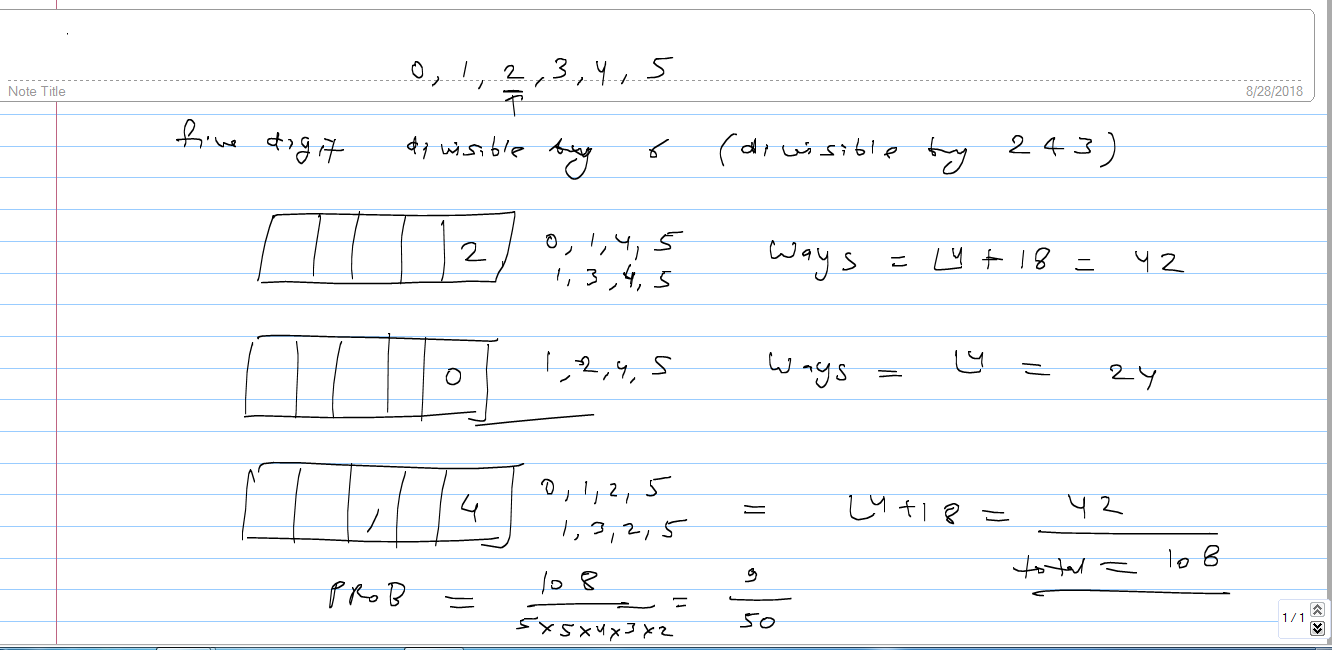# how to find whether the given curve has vertical tangent at given point(x,y)

4 years ago

A vertical tangent touches the curve at a point where the gradient (slope) of the curve is infinite and undefined.

To find the vertical tangent in calculus and the gradient of a curve, you need to differentiate the function that describes the curve. For example, if the curve is y = √(x – 2), then differentiating this equation to find dy/dx will give you the gradient of the curve. Next, find a value of x that makes dy/dx infinite. The vertical tangent of the curve is a vertical line at this value of x.Deepak Kumar Shringi